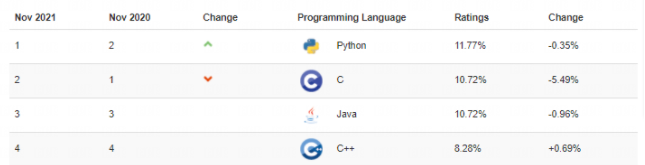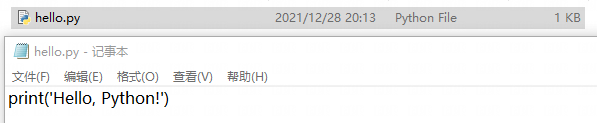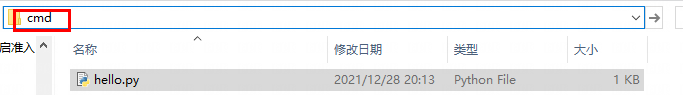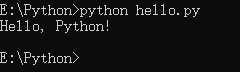# 《Python入门到精通》Python基础语法<font color="orange">「作者主页」：</font>士别三日wyx
此文章已录入专栏<font color="greeg">《Python入门到精通》</font>
2021最新版Python小白教程，针对0基础小白和基础薄弱的伙伴学习``C:\Users\zhangsan>python --version``

``Python 3.8.6``

``print('Hello, Python!')``第二步： 在文件所在目录的地址栏中输入cmd（当前目录打开cmd）恭喜，你已经编写并执行了第一个python程序，到官网下载开发工具 PyCharm

``https://www.jetbrains.com/pycharm/download/#section=windows -- 官网``

Python中使用缩进来指示代码块，即代码行开头的「空格」

``````if 2 > 1:
print('Hello, Python!')``````

``Hello, Python!``
``````if 2 > 1:
print('Hello, Python!')``````

``````File "E:/data/PrCharm/test/test.py", line 2
print('Hello, Python!')
^
IndentationError: expected an indented block
# 缩进错误：此处需要缩进``````

## 3.1 单行注释

Python的注释以#开头

``````# 注释不会被执行
print('Hello, Python!')``````

``Hello, Python!``

## 3.2 字符串注释

「未分配」给变量的字符串不会被执行，可当做注释使用

``````'字符串注释'
print('Hello, Python!')``````

``Hello, Python!``

## 3.3 多行注释

``````''' 多行注释 多行注释 '''
print('Hello, Python!')``````

``Hello, Python!``

## 4.1 创建变量

Python的变量在被赋值时「创建」，不需要声明变量

``````text = 5
print(text)``````

``5``

## 4.2 变量类型

Python会根据变量内容自动确定「变量类型」，不需要「声明」变量类型，可随意「修改」数据类型

``````text = 5
text = '张三'
print(text)``````

``张三``

## 4.3 变量命名规则

1. 变量名只能包含字母、数字和下划线（A-z、0-9 和 _）
2. 变量名必须以字母或下划线开头，不能以数字开头
3. 变量名区分大小写（如text 和 Text 是不同的变量）

## 4.4 多变量赋值

Python可以同时为「多个变量赋值」，变量与值的「位置」一一对应

``````x, y, z = '张三', '李四', '王五'
print(x, y, z)``````

``张三 李四 王五``
``````x = y = z = '张三'
print(x, y, z)``````

``张三 张三 张三``

## 4.5 全局变量

``````name = '张三' # 全局变量
def hello():
print(name)
hello()``````

``张三``

## 4.6 局部变量

``````def hello():
name = '张三' # 局部变量
print(name)

hello()``````

``张三``
``````def hello():
name = '张三' # 局部变量

hello()
print(name)``````

``````Traceback (most recent call last):
File "E:/data/PrCharm/test/test.py", line 4, in <module>
print(name)
NameError: name 'name' is not defined
# 名称错误，变量‘name’没有被定义``````

## 4.7 global关键字

global可以将局部变量更改为全局变量

``````def hello():
global name # 定义全局变量
name = '张三'  # 赋值

hello()
print(name)``````

``张三``
``````def hello():
name = '张三'  # 赋值
global name  # 定义全局变量

hello()
print(name)``````

``````File "E:/data/PrCharm/test/test.py", line 3
global name  # 定义全局变量
^
SyntaxError: name 'name' is assigned to before global declaration
# 语法错误：变量‘name’在全局声明之前被赋值``````

-- 展开阅读全文 --

Redis底层数据结构--跳跃表
« 上一篇 04-28
BUUCTF Web [强网杯 2019]随便注